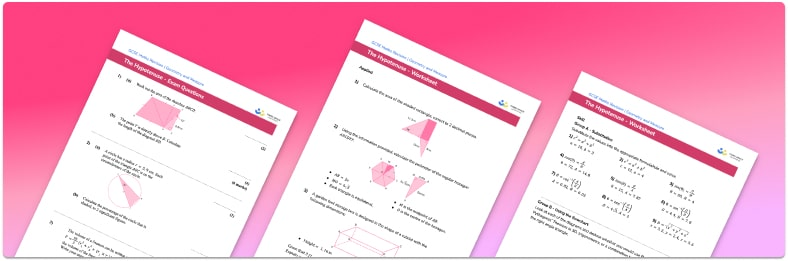# Hypotenuse Worksheet• Section 1 of the The Hypotenuse worksheet contains 27 skills-based questions, in 3 groups to support differentiation
• Section 2 contains 3 applied The Hypotenuse questions with a mix of worded problems and deeper problem solving questions
• Section 3 contains 3 foundation and higher level GCSE exam style The Hypotenuse questions
• Answers and a mark scheme for all The Hypotenuse questions
• Follows variation theory with plenty of opportunities for students to work independently at their own level
• All questions created by fully qualified expert secondary maths teachers
• Suitable for GCSE maths revision for AQA, OCR and Edexcel exam boards

• This field is for validation purposes and should be left unchanged.

You can unsubscribe at any time (each email we send will contain an easy way to unsubscribe). To find out more about how we use your data, see our privacy policy.

### Hypotenuse at a glance

The hypotenuse is the name of the longest side of a right-angled triangle, it is the side that is opposite the right angle. It is one of the three sides of the triangle with the other two called the opposite and the adjacent.

Finding the hypotenuse of a triangle is a common exam question and is often asked as part of a word problem. In order to calculate the length of the hypotenuse when we know the other two sides we can use Pythagoras. The pythagorean theorem involves the lengths of the side of a right-angled triangle and states that the sum of the squares of the two shorter sides is equal to the square of the longest side (the square of the hypotenuse). In order to work out the missing length of the missing side we can square the lengths of the shorter sides, add them together and then square root the answer to work out the hypotenuse.

We can work out the length of an unknown side that is shorter than the hypotenuse by squaring the longest side and squaring the other shorter side, subtracting them and then square rooting the answer.

The side lengths of right-angled triangles can be decimals or integers. When they are whole numbers the three lengths are known as a pythagorean triple.

Looking forward, students can then progress to additional geometry worksheets, for example a 3D Pythagoras worksheet or .For more teaching and learning support on Geometry our GCSE maths lessons provide step by step support for all GCSE maths concepts.

## Do you have KS4 students who need more focused attention to succeed at GCSE?There will be students in your class who require individual attention to help them succeed in their maths GCSEs. In a class of 30, it’s not always easy to provide.

Help your students feel confident with exam-style questions and the strategies they’ll need to answer them correctly with our dedicated GCSE maths revision programme.

Lessons are selected to provide support where each student needs it most, and specially-trained GCSE maths tutors adapt the pitch and pace of each lesson. This ensures a personalised revision programme that raises grades and boosts confidence.

Find out more